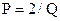Chapter 5, Problem 34P### Principles of Economics 2e

2nd Edition
Steven A. Greenlaw; David Shapiro
ISBN: 9781947172364

#### Solutions

Chapter
Section### Principles of Economics 2e

2nd Edition
Steven A. Greenlaw; David Shapiro
ISBN: 9781947172364
Textbook Problem

# The equation for a demand curve isWhat is the elasticity of demand as price falls from 5 to 4? What is the elasticity of demand as the price falls from 9 to 8? Would you expect these answers to be the same?

To determine

Calculate the elasticity of demand for the following demand function when price falls from 5 to 4 and 9 to 8. Also tell is the answer same for both cases?

P =2Q.

Explanation

We will first find the quantity demanded at price $5 P = 2Q5 = 2QQ = 0.40Q = 0.40 units at P =$5

Now, we will find quantity demanded at price $4 P = 2Q4 = 2QQ = 0.50Q = 0.50 units at P =$4

Now, we can calculate the elasticity of demand with the following formula:

e = ΔQΔP×PQ

e = 0.500.4054×50.40e = 0.101×50.40e = 1.25

Therefore, the elasticity of demand is 1.25 when price falls from $5 to$4.

Now we will calculate the elasticity of demand when price falls from $9 to$8.

We will first find the quantity demanded at price \$9

P = 2Q9 = 2QQ = 0

### Still sussing out bartleby?

Check out a sample textbook solution.

See a sample solution

#### The Solution to Your Study Problems

Bartleby provides explanations to thousands of textbook problems written by our experts, many with advanced degrees!

Get Started

#### Find more solutions based on key concepts